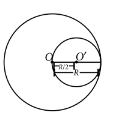Moment of intertia
Question

# From a disc of radius $R$and mass $M$ a circular hole of diameter$R$ whose rim passes through the centre is cut. What is the moment of inertia of the remaining part of the disc about a perpendicular axis, passing through the centre?

Moderate
Solution

##Mass of removed portion of disc,${M}^{\text{'}}=\frac{M}{\pi {R}^{2}}×\pi {\left(\frac{R}{2}\right)}^{2}=\frac{M}{4}$Moment of inertia of removed portion about an axis passing through centre of disc O and perpendicular to the plane of disc,${I}_{O}^{\text{'}}={I}_{{O}^{\text{'}}}+{M}^{\text{'}}{d}^{2}$$=\frac{1}{2}×\frac{M}{4}×{\left(\frac{R}{2}\right)}^{2}+\frac{M}{4}×{\left(\frac{R}{2}\right)}^{2}=\frac{M{R}^{2}}{32}+\frac{M{R}^{2}}{16}=\frac{3M{R}^{2}}{32}$When portion of disc would not have been removed, the moment of inertia of complete disc about centre O is${I}_{O}=\frac{1}{2}M{R}^{2}$ So, moment of inertia of the disc with removed portion is $I={I}_{O}-{I}_{O}^{\text{'}}=\frac{1}{2}M{R}^{2}-\frac{3M{R}^{2}}{32}=\frac{13M{R}^{2}}{32}$

Get Instant Solutions